Courses

# Test: Overlapping SETS- 1

## 15 Questions MCQ Test Quantitative Aptitude for GMAT | Test: Overlapping SETS- 1

Description
This mock test of Test: Overlapping SETS- 1 for GMAT helps you for every GMAT entrance exam. This contains 15 Multiple Choice Questions for GMAT Test: Overlapping SETS- 1 (mcq) to study with solutions a complete question bank. The solved questions answers in this Test: Overlapping SETS- 1 quiz give you a good mix of easy questions and tough questions. GMAT students definitely take this Test: Overlapping SETS- 1 exercise for a better result in the exam. You can find other Test: Overlapping SETS- 1 extra questions, long questions & short questions for GMAT on EduRev as well by searching above.
QUESTION: 1

### Of the films Empty Set Studios released last year, 60% were comedies and the rest were horror films. 75% of the comedies were profitable, but 75% of the horror moves were unprofitable. If the studio made a total of 40 films, and broke even on none of them, how many of their films were profitable?

Solution:

For an overlapping sets problem it is best to use a double set matrix to organize the information and solve.  Fill in the information in the order in which it is given.

Of the films Empty Set Studios released last year, 60% were comedies and the rest were horror films.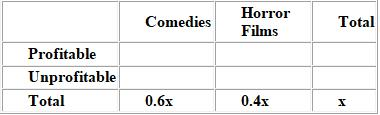75% of the comedies were profitable, but 75% of the horror moves were unprofitable.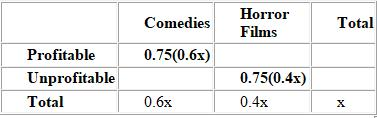If the studio made a total of 40 films...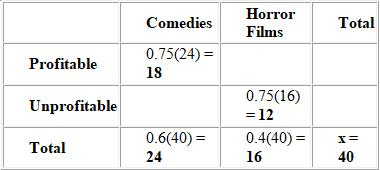Since each row and each column must sum up to the Total value, we can fill in the remaining boxes.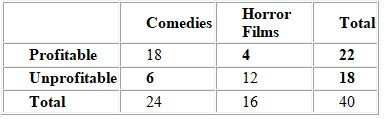The problem seeks the total number of profitable films, which is 22.

QUESTION: 2

### At a certain hospital, 75% of the interns receive fewer than 6 hours of sleep and report feeling tired during their shifts. At the same time, 70% of the interns who receive 6 or more hours of sleep report no feelings of tiredness. If 80% of the interns receive fewer than 6 hours of sleep, what percent of the interns report no feelings of tiredness during their shifts?

Solution:

For an overlapping sets problem we can use a double-set matrix to organize our information and solve.  Because the values are in percents, we can assign a value of 100 for the total number of interns at the hospital.  Then, carefully fill in the matrix based on the information provided in the problem.  The matrix below details this information.  Notice that the variable x is used to detail the number of interns who receive 6 or more hours of sleep, 70% of whom reported no feelings of tiredness.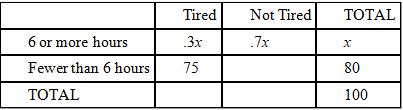In a double-set matrix, the sum of the first two rows equals the third and the sum of the first two columns equals the third. Thus, the boldfaced entries below were derived using the above matrix.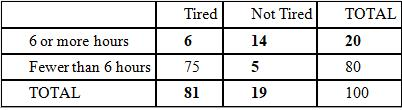We were asked to find the percentage of interns who reported no feelings of tiredness, or 19% of the interns.

QUESTION: 3

### All of the students of Music High School are in the band, the orchestra, or both. 80 percent of the students are in only one group. There are 119 students in the band. If 50 percent of the students are in the band only, how many students are in the orchestra only?

Solution:

This is an overlapping sets problem concerning two groups (students in either band or orchestra) and the overlap between them (students in both band and orchestra).

If the problem gave information about the students only in terms of percents, then a smart number to use for the total number of students would be 100. However, this problem gives an actual number of students (“there are 119 students in the band”) in addition to the percentages given. Therefore, we cannot assume that the total number of students is 100.

Instead, first do the problem in terms of percents. There are three types of students: those in band, those in orchestra, and those in both. 80% of the students are in only one group. Thus, 20% of the students are in both groups. 50% of the students are in the band only. We can use those two figures to determine the percentage of students left over: 100% - 20% - 50% = 30% of the students are in the orchestra only.

Great - so 30% of the students are in the orchestra only. But although 30 is an answer choice, watch out! The question doesn't ask for the percentage of students in the orchestra only, it asks for the number of students in the orchestra only. We must figure out how many students are in Music High School altogether.

The question tells us that 119 students are in the band. We know that 70% of the students are in the band: 50% in band only, plus 20% in both band and orchestra. If we let x be the total number of students, then 119 students are 70% of x, or 119 = .7x. Therefore, x = 119 / .7 = 170 students total.

The number of students in the orchestra only is 30% of 170, or .3 × 170 = 51.

QUESTION: 4

How many attendees are at a convention if 150 of the attendees are neither female nor students, one-sixth of
the attendees are female students, two-thirds of the attendees are female, and one-third of the attendees are
students?

Solution:

For an overlapping set problem we can use a double-set matrix to organize our information and solve. Let's call P the number of people at the convention. The boldface entries in the matrix below were given in the question. For example, we are told that one sixth of the attendees are female students, so we put a value of P/6 in the female students cell.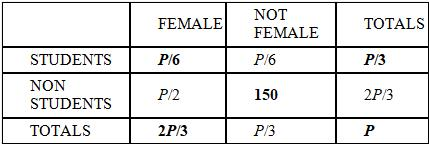The non-boldfaced entries can be derived using simple equations that involve the numbers in one of the "total" cells. Let's look at the "Female" column as an example. Since we know the number of female students (P/6) and we know the total number of females (2P/3), we can set up an equation to find the value of female non-students:

P/6 + Female Non Students = 2P/3.
Solving this equation yields: Female Non Students = 2P/3 – P/6 = P/2.

By solving the equation derived from the "NOT FEMALE" column, we can determine a value for P.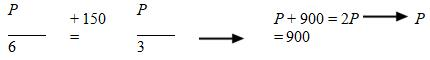The correct answer is E.

QUESTION: 5

Eighty percent of the lights at Hotel California are on at 8 p.m. a certain evening. However, forty percent of
the lights that are supposed to be off are actually on and ten percent of the lights that are supposed to be on
are actually off. What percent of the lights that are on are supposed to be off?

Solution:

For an overlapping set problem we can use a double-set matrix to organize our information and solve.  Because the values here are percents, we can assign a value of 100 to the total number of lights at Hotel California. The information given to us in the question is shown in the matrix in boldface.  An x was assigned to the lights that were “Supposed To Be Off” since the values given in the problem reference that amount.  The other values were filled in using the fact that in a double-set matrix the sum of the first two rows equals the third and the sum of the first two columns equals the third.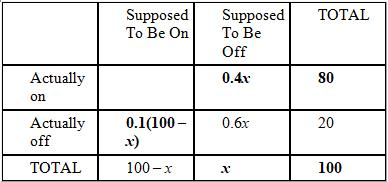Using the relationships inherent in the matrix, we see that:
0.1(100 – x) + 0.6x = 20
10 – 0.1x + 0.6x = 20
0.5x = 10 so x = 20

We can now fill in the matrix with values: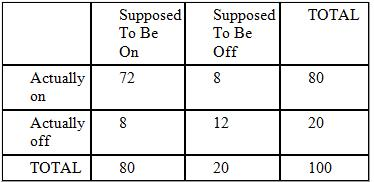Of the 80 lights that are actually on, 8, or 10% percent, are supposed to be off.

QUESTION: 6

Of the 645 speckled trout in a certain fishery that contains only speckled and rainbow trout, the number of
males is 45 more than twice the number of females. If the ratio of female speckled trout to male rainbow
trout is 4:3 and the ratio of male rainbow trout to all trout is 3:20, how many female rainbow trout are there?

Solution:

This question involves overlapping sets so we can employ a double-set matrix to help us. The two sets are speckled/rainbow and male/female. We can fill in 645 for the total number of total speckled trout based on the first sentence. Also, we can assign a variable, x, for female speckled trout and the expression 2x + 45 for male speckled trout, also based on the first sentence.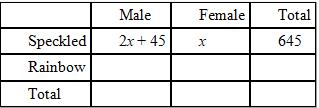We can solve for x with the following equation: 3x + 45 = 645. Therefore, x = 200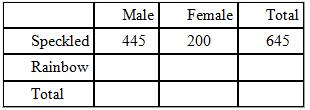If the ratio of female speckled trout to male rainbow trout is 4:3, then there must be 150 male rainbow trout. We can easily solve for this with the below proportion where y represents male rainbow trout: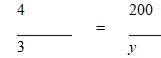Therefore, y = 150.  Also, if the ratio of male rainbow trout to all trout is 3:20, then there must be 1000 total trout using the below proportion, where z represents all trout: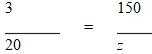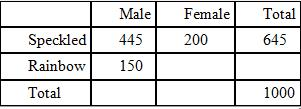Now we can just fill in the empty boxes to get the number of female rainbow trout.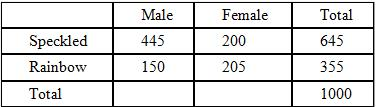QUESTION: 7

30% of major airline companies equip their planes with wireless internet access. 70% of major airlines offer
passengers free on-board snacks. What is the greatest possible percentage of major airline companies that
offer both wireless internet and free on-board snacks?

Solution:

Begin by constructing a double-set matrix and filling in the information given in the problem. Assume there are 100 major airline companies in total since this is an easy number to work with when dealing with percent problems.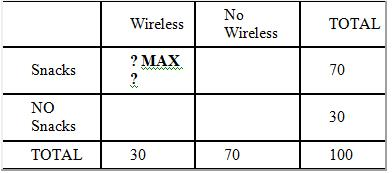Notice that we are trying to maximize the cell where wireless intersects with snacks. What is the maximum possible value we could put in this cell. Since the total of the snacks row is 70 and the total of the wireless column is 30, it is clear that 30 is the limiting number. The maximum value we can put in the wireless-snacks cell is therefore 30. We can put 30 in this cell and then complete the rest of the matrix to ensure that all the sums will work correctly.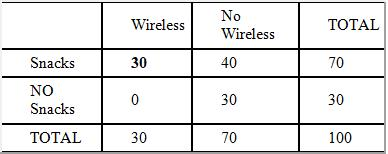QUESTION: 8

In country Z, 10% of the people do not have a university diploma but have the job of their choice, and 25%
of the people who do not have the job of their choice have a university diploma. If 40% of the people have
the job of their choice, what percent of the people have a university diploma?

Solution:

For an overlapping set problem we can use a double-set matrix to organize our information and solve. Because the given values are all percentages, we can assign a value of 100 to the total number of people in country Z. The matrix is filled out below based on the information provided in the question.
The first sentence tells us that 10% of all of the people do have their job of choice but do not have a diploma, so we can enter a 10 into the relevant box, below. The second sentence tells us that 25% of those who do not have their job of choice have a diploma.  We don't know how many people do not have their job of choice, so we enter a variable (in this case, x) into that box.  Now we can enter 25% of those people, or 0.25x, into the relevant box, below.  Finally, we're told that 40% of all of the people have their job of choice.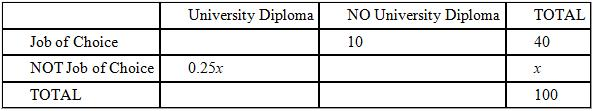In a double-set matrix, the sum of the first two rows equals the third and the sum of the first two columns equals the third. Thus, the boldfaced entries below were derived using relationships (for example: 40 + x = 100, therefore x = 60.  0.25 × 60 = 15. And so on.).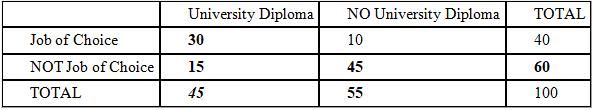We were asked to find the percent of the people who have a university diploma, or 45%.

QUESTION: 9

Seventy percent of the 800 students in School T are male. At least ten percent of the female students in
School T participate in a sport. Fewer than thirty percent of the male students in School T do not participate
in a sport. What is the maximum possible number of students in School T who do not participate in a sport?

Solution:

This is a problem that involves two overlapping sets so it can be solved using a double-set matrix. The problem tells us that there are 800 total students of whom 70% or 560 are male. This means that 240 are female and we can begin filling in the matrix as follows: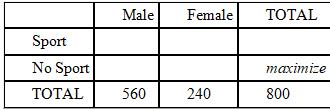The question asks us to MAXIMIZE the total number of students who do NOT participate in a sport. In order to maximize this total, we will need to maximize the number of females who do NOT participate in and the number of males who do NOT participate in a sport.
The problem states that at least 10% of the female students, or 24 female students, participate in a sport. This leaves 216 female students who may or may not participate in a sport. Since we want to maximize the number of female students who do NOT participate in a sport, we will assume that all 216 of these remaining female students do not participate in a sport.
The problem states that fewer than 30% of the male students do NOT participate in a sport. Thus, fewer than 168 male students (30% of 560) do NOT participate in a sport. Thus anywhere from 0 to 167 male students do NOT participate in a sport. Since we want to maximize the number of male students who do NOT participate in a sport, we will assume that 167 male students do NOT participate in a sport. This leaves 393 male students who do participate in a sport.
Thus, our matrix can now be completed as follows: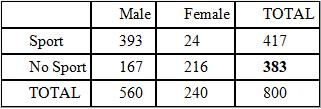Therefore, the maximum possible number of students in School T who do not participate in a sport is 383.

QUESTION: 10

75% of the guestrooms at the Stagecoach Inn have a queen-sized bed, and each of the remaining rooms has
a king-sized bed. Of the non-smoking rooms, 60% have a queen-sized bed. If 10% of the rooms at the
Stagecoach Inn are non-smoking rooms with king-sized beds, what percentage of the rooms permit smoking?

Solution:

This is an overlapping sets problem, which can be solved most efficiently by using a double set matrix.  Our first step in using the double set matrix is to fill in the information given in the question.  Because there are no real values given in the question, the problem can be solved more easily using 'smart numbers'; in this case, we can assume the total number of rooms to be 100 since we are dealing with percentages.  With this assumption, we can fill the following information into our matrix:

There are 100 rooms total at the Stagecoach Inn.

Of those 100 rooms, 75 have a queen-sized bed, while 25 have a king-sized bed.

Of the non-smoking rooms (let's call this unknown n), 60% or .6n have queen-sized beds.

10 rooms are non-smoking with king-sized beds.

Let's fill this information into the double set matrix, including the variable n for the value we need to solve the problem: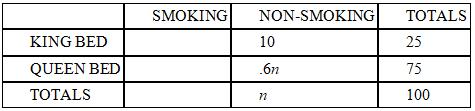In a double-set matrix, the first two rows sum to the third, and the first two columns sum to the third. We can therefore solve for n using basic algebra:

10 + .6n = n
10 = .4n
n = 25
We could solve for the remaining empty fields, but this is unnecessary work.  Observe that the total number of smoking rooms equals 100 – n = 100 – 25 = 75.  Recall that we are working with smart numbers that represent percentages, so 75% of the rooms at the Stagecoach Inn permit smoking.

QUESTION: 11

At the end of the day, February 14th, a florist had 120 roses left in his shop, all of which were red, white or
pink in color and either long or short-stemmed. A third of the roses were short-stemmed, 20 of which were
white and 15 of which were pink. The percentage of pink roses that were short-stemmed equaled the
percentage of red roses that were short-stemmed. If none of the long-stemmed roses were white, what
percentage of the long-stemmed roses were red?

Solution:

For an overlapping set problem we can use a double-set matrix to organize our information and solve.  The boldfaced values were given in the question. The non-boldfaced values were derived using the fact that in a double-set matrix, the sum of the first two rows equals the third and the sum of the first two columns equals the third.  The variable p was used for the total number of pink roses, so that the total number of pink and red roses could be solved using the additional information given in the question.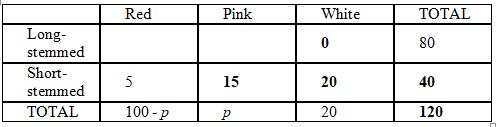The question states that the percentage of red roses that are short-stemmed is equal to the percentage of pink roses that are short stemmed, so we can set up the following proportion: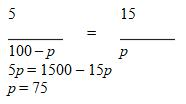This means that there are a total of 75 pink roses and 25 red roses.   Now we can fill out the rest of the double-set matrix: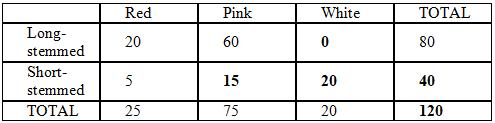Now we can answer the question.  20 of the 80 long-stemmed roses are red, or 20/80 = 25%.

QUESTION: 12

Some of the people in Town X are left-handed, some are tall, some are both, and some are neither. In Town
Y, three times as many people are left-handed as are left-handed in Town X, three times as many people are
tall as are tall in Town X, three times as many people are both as are both in Town X, but no one is neither. If
the total number of people in Town X is four times greater than the total number of people in Town Y, which
of the following could be the number of people in Town X who are neither left-handed nor tall?

Solution:

The best way to approach this question is to construct a matrix for each town.  Let's start with Town X.  Since we are not given any values, we will insert unknowns into the matrix: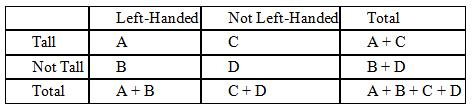Now let's create a matrix for Town Y, using the information from the question and the unknowns from the matrix for Town X: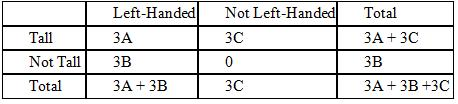Since we know that the total number of people in Town X is four times greater than the total number of people in Town Y, we can construct and simplify the following equation: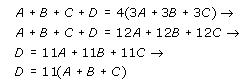Since D represents the number of people in Town X who are neither tall nor left-handed, we know that the correct answer must be a multiple of 11.  The only answer choice that is a multiple of 11 is 143  .

QUESTION: 13

The waiter at an expensive restaurant has noticed that 60% of the couples order dessert and coffee.
However, 20% of the couples who order dessert don't order coffee. What is the probability that the next
couple the waiter seats will not order dessert?

Solution:

You can solve this problem with a matrix. Since the total number of diners is unknown and not important in solving the problem, work with a hypothetical total of 100 couples. Since you are dealing with percentages, 100 will make the math easier.

Set up the matrix as shown below: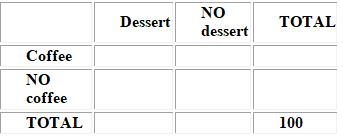Since you know that 60% of the couples order BOTH dessert and coffee, you can enter that number into the matrix in the upper left cell.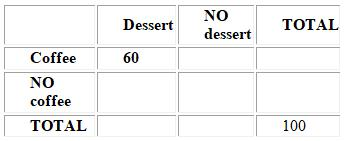The next useful piece of information is that 20% of the couples who order dessert don't order coffee. But be careful! The problem does not say that 20% of the total diners order dessert and don't order coffee, so you CANNOT fill in 40 under "dessert, no coffee" (first column, middle row). Instead, you are told that 20% of the couples who order dessert don't order coffee.

Let x = total number of couples who order dessert. Therefore you can fill in .2x for the number of couples who order dessert but no coffee.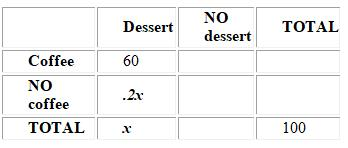Set up an equation to represent the couples that order dessert and solve:

75% of all couples order dessert. Therefore, there is only a 25% chance that the next couple the maitre 'd seats will not order dessert. The correct answer is B.

QUESTION: 14

50% of the apartments in a certain building have windows and hardwood floors. 25% of the apartments
without windows have hardwood floors. If 40% of the apartments do not have hardwood floors, what
percent of the apartments with windows have hardwood floors?

Solution:

This problem involves two sets:
Set 1: Apartments with windows / Apartments without windows
Set 2: Apartments with hardwood floors / Apartments without hardwood floors.

It is easiest to organize two-set problems by using a matrix as follows: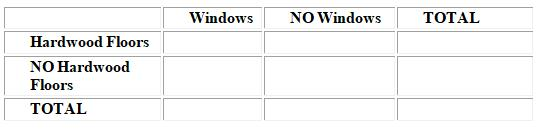The problem is difficult for two reasons. First, it uses percents instead of real numbers. Second, it involves complicated and subtle wording.

Let's attack the first difficulty by converting all of the percentages into REAL numbers. To do this, let's say that there are 100 total apartments in the building. This is the first number we can put into our matrix. The absolute total is placed in the lower right hand corner of the matrix as follows: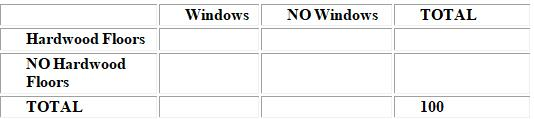Next, we will attack the complex wording by reading each piece of information separately, and filling in the matrix accordingly.

Information: 50% of the apartments in a certain building have windows and hardwood floors. Thus, 50 of the 100 apartments have BOTH windows and hardwood floors. This number is now added to the matrix: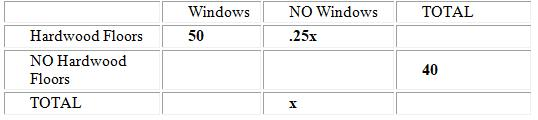Before answering the question, we must complete the matrix. To do this, fill in the numbers that yield the given totals. First, we see that there must be be 60 total apartments with Hardwood Floors (since 60 + 40 = 100) Using this information, we can solve for x by creating an equation for the first row of the matrix: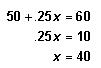Now we put these numbers in the matrix: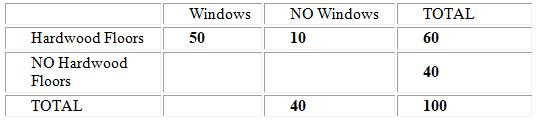Finally, we can fill in the rest of the matrix: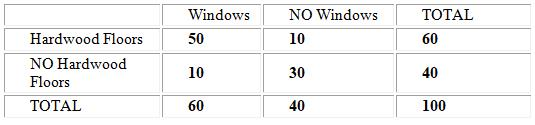We now return to the question: What percent of the apartments with windows have hardwood floors?

Again, pay very careful attention to the subtle wording. The question does NOT ask for the percentage of TOTAL apartments that have windows and hardwood floors. It asks what percent OF the apartments with windows have hardwood floors. Since there are 60 apartments with windows, and 50 of these have hardwood floors, the percentage is calculated as follows: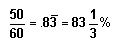Thus, the correct answer is E.

QUESTION: 15

A farmer has an apple orchard consisting of Fuji and Gala apple trees. Due to high winds this year 10% of his
trees cross pollinated. The number of his trees that are pure Fuji plus the cross-pollinated ones totals 187,
while 3/4 of all his trees are pure Fuji. How many of his trees are pure Gala?

Solution:

This problem can be solved using a set of three equations with three unknowns. We'll use the following definitions:

Let F = the number of Fuji trees
Let G = the number of Gala trees
Let C = the number of cross pollinated trees

10% of his trees cross pollinated
C = 0.1(F + G + C)
10C = F + G + C
9C = F + G

The pure Fujis plus the cross pollinated ones total 187
(4) F + C = 187

3/4 of his trees are pure Fuji
(5) F = (3/4)(F + G + C)
(6) 4F = 3F + 3G + 3C
(7) F = 3G + 3C

Substituting the value of F from equation (7) into equation (3) gives us:

(8) 9C = (3G + 3C) + G
(9) 6C = 4G
(10) 12C = 8G

Substituting the value of F from equation (7) into equation (4) gives us:
(11) (3G + 3C) + C = 187
(12) 3G + 4C = 187
(13) 9G + 12C = 561

Substituting equation (10) into (13) gives:
(14) 9G + 8G = 561
(15) 17G = 561
(16) G = 33

So the farmer has 33 trees that are pure Gala.